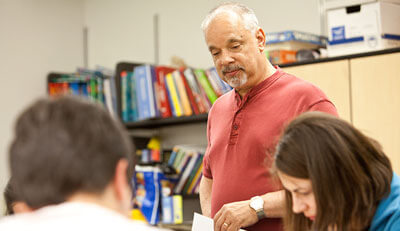# Calculus II

The second course in calculus, MAT 1235, emphasizes integral calculus of functions of one variable. The primary aims of the course are to help you develop new problem-solving and critical-reasoning skills and to prepare you for further study in mathematics, the physical sciences, or engineering.

By the end of the course, you should be able to:

• Evaluate definite and indefinite integrals.
• Interpret definite integrals as accumulations of rates of change and as Riemann sums.
• Explain and apply the Fundamental Theorem of Calculus.
• Recognize the difference between definite and indefinite integrals.
• Apply integration to several types of physical problems.
• Differentiate, integrate, and solve problems with exponential, logarithmic, and inverse trigonometric functions.
• Compute complicated integrals using a combination of substitutions, algebraic and trigonometric manipulation, partial fractions, and parts.
• Recognize and compute improper integrals.
• Compute areas, volumes, and arc lengths.
• Recognize and solve some basic differential equations, including separable equations, logistic equations, and first order linear equations.
• Use Maple effectively to explore and solve calculus problems.
• Analyze and solve complex problems.
• Provide clear written explanations of the ideas behind key concepts from the course.

You should also gain an increased appreciation of mathematics as part of the language of science and as a study in itself.#### Why I Teach at SPU

##### Russ Killingsworth, Assistant Professor of Mathematics

“I teach at SPU because of the emphasis on a liberal arts education, framed in an evangelical context. Here we are able to see and experience mathematics through the eyes of our Creator.”